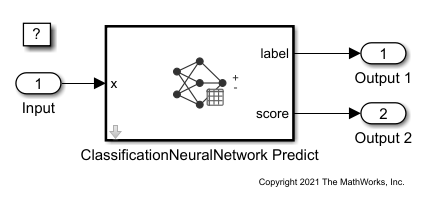# Predict Class Labels Using ClassificationNeuralNetwork Predict Block

This example shows how to use the ClassificationNeuralNetwork Predict block for label prediction in Simulink®. The block accepts an observation (predictor data) and returns the predicted class label and class score for the observation using the trained neural network classification model.

### Train Neural Network Classifier

Train a neural network classifier, and assess the performance of the classifier on a test set.

Load the `humanactivity` data set. This data set contains 24,075 observations of five physical human activities: Sitting, Standing, Walking, Running, and Dancing. Each observation has 60 features extracted from acceleration data measured by smartphone accelerometer sensors.

`load humanactivity`

Create the predictor `X` as a numeric matrix that contains 60 features for 24,075 observations. Create the class labels `Y` as a numeric vector that contains the activity IDs in integers: 1, 2, 3, 4, and 5 representing Sitting, Standing, Walking, Running, and Dancing, respectively.

```X = feat; Y = actid;```

Randomly partition observations into a training set and a test set with stratification, using the class information in Y. Use approximately 80% of the observations to train a neural network model, and 20% of the observations to test the performance of the trained model on new data.

```rng("default") % For reproducibility of the partition cv = cvpartition(Y,"Holdout",0.20);```

Extract the training and test indices.

```trainingInds = training(cv); testInds = test(cv);```

Specify the training and test data sets.

```XTrain = X(trainingInds,:); YTrain = Y(trainingInds); XTest = X(testInds,:); YTest = Y(testInds);```

Train a neural network classifier by passing the training data `XTrain` and `YTrain` to the `fitcnet` function. Specify 40 outputs for the first fully connected layer and 20 outputs for the second fully connected layer.

`nnetMdl = fitcnet(XTrain,YTrain,"LayerSizes",[40 20])`
```nnetMdl = ClassificationNeuralNetwork ResponseName: 'Y' CategoricalPredictors: [] ClassNames: [1 2 3 4 5] ScoreTransform: 'none' NumObservations: 19260 LayerSizes: [40 20] Activations: 'relu' OutputLayerActivation: 'softmax' Solver: 'LBFGS' ConvergenceInfo: [1x1 struct] TrainingHistory: [1000x7 table] ```

`nnetMdl` is a trained `ClassificationNeuralNetwork` model. You can use dot notation to access the properties of `nnetMdl`. For example, you can specify `nnetMdl.TrainingHistory` to get more information about the training history of the neural network model.

Evaluate the performance of the classifier on the test set by computing the test set classification accuracy.

```testError = loss(nnetMdl,XTest,YTest,"LossFun","classiferror"); testAccuracy = 1 - testError```
```testAccuracy = 0.9819 ```

This example provides the Simulink model `slexClassificationNeuralNetworkPredictExample.slx`, which includes the ClassificationNeuralNetwork Predict block. You can open the Simulink model or create a new model as described in this section.

#### Open Provided Model

Open the Simulink model `slexClassificationNeuralNetworkPredictExample.slx`.

```SimMdlName = 'slexClassificationNeuralNetworkPredictExample'; open_system(SimMdlName)```If you open the Simulink model, then the software runs the code in the `PreLoadFcn` callback function before loading the Simulink model. The `PreLoadFcn` callback function of `slexClassificationNeuralNetworkPredictExample` includes code to check if your workspace contains the `nnetMdl` variable for the trained model. If the workspace does not contain the variable, `PreLoadFcn` loads the sample data, trains the neural network model, and creates an input signal for the Simulink model. To view the callback function, in the Setup section on the Modeling tab, click Model Settings and select Model Properties. Then, on the Callbacks tab, select the `PreLoadFcn` callback function in the Model callbacks pane.

#### Create New Model

Instead of opening the model provided, you can create a new model. To create a new Simulink model, open the Blank Model template and add the ClassificationNeuralNetwork Predict block. Add the Inport and Outport blocks and connect them to the ClassificationNeuralNetwork Predict block.

Double-click the ClassificationNeuralNetwork Predict block to open the Block Parameters dialog box. You can specify the name of a workspace variable that contains the trained neural network model. The default variable name is `nnetMdl`. Click the Refresh button. The Trained Machine Learning Model section of the dialog box displays the options used to train the model `nnetMdl`. Select the Add output port for predicted class scores check box to add the second output port score.The ClassificationNeuralNetwork Predict block expects an observation containing 60 predictor values. Double-click the Inport block, and set the Port dimensions to 60 on the Signal Attributes tab.

Create an input signal in the form of a structure array for the Simulink model. The structure array must contain these fields:

• `time` — The points in time at which the observations enter the model. The orientation must correspond to the observations in the predictor data. In this example, `time` must be a column vector.

• `signals` — A 1-by-1 structure array describing the input data and containing the fields `values` and `dimensions`, where `values` is a matrix of predictor data, and `dimensions` is the number of predictor variables.

Create an appropriate structure array for future human activity.

```activityInput.time = (0:length(YTest)-1)'; activityInput.signals(1).values = XTest; activityInput.signals(1).dimensions = size(XTest,2);```

Import the signal data from the workspace:

• Open the Configuration Parameters dialog box. On the Modeling tab, click Model Settings.

• In the Data Import/Export pane, select the Input check box and enter `activityInput` in the adjacent text box.

• In the Solver pane, under Simulation time, set Stop time to `activityInput.time(end)`. Under Solver selection, set Type to `Fixed-step`, and set Solver to `discrete (no continuous states)`.

`sim(SimMdlName);`